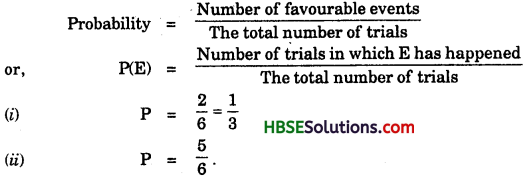# HBSE 7th Class Maths Solutions Chapter 3 Data Handling Ex 3.4

Haryana State Board HBSE 7th Class Maths Solutions Chapter 3 Data Handling Ex 3.4 Textbook Exercise Questions and Answers.

## Haryana Board 7th Class Maths Solutions Chapter 3 Data Handling Exercise 3.4

Question 1.
Tell whether the following is certain to happen, impossible, can happen but not certain.
(i) You are older today than yesterday.
(ii) A tossed coin will land heads up.
(iii) A die when tossed shall land up with 8 on top.
(iv) The next traffic light seen will be green.
(v) Tomorrow will be a cloudy day.
Solution:
(i) 0, (ii) between 0 and 1, (iii) 0, (iv) between 0 and 1, (v) between 0 and 1.

Question 2.
There are 6 marbles in a box can with numbers from 1 to 6 marked on each ofthem.
(i) What is the probability of drawing marble with number 2 ?
(ii) What is the probability of drawing marble with number 5 ?
Solution:Question 3.
A coin is flipped to decide which team starts the game. What is the probability that your team will start ?
Solution:
A coin has one head and one tail
∴ P = $$\frac{1}{2}$$

Question 4.
A box contains pairs of socks of two colours (black and white) I have picked out a white sock. I pick out one more with my eyes closed. What is the probability that it will make a pair ?
Solution:
A box contains socks of two colours black and white.
∴ P = $$\frac{1}{2}$$